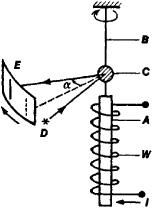# Einstein-de Haas Effect

Also found in: Wikipedia.

## Einstein-de Haas effect

[′īn‚stīn də′häs i‚fekt]
(electromagnetism)
A freely suspended body consisting of a ferromagnetic material acquires a rotation when its magnetization changes.
McGraw-Hill Dictionary of Scientific & Technical Terms, 6E, Copyright © 2003 by The McGraw-Hill Companies, Inc.
The following article is from The Great Soviet Encyclopedia (1979). It might be outdated or ideologically biased.

## Einstein-de Haas Effect

an effect whereby a body (a ferromagnetic material), when magnetized along some axis, acquires an angular impulse relative to the axis, which is proportional to the acquired magnetization. The effect was experimentally discovered and theoretically explained in 1915 by Albert Einstein and the Dutch physicist W. de Haas.Figure 1. Diagram of apparatus for measuring the Einstein-de Haas effect: (A) sample, (B) elastic thread of suspension device, (C) mirror, (a) angle of rotation of sample, registered from the change In position of the reflected light ray, (D) light source, (E) scale, (W) magnetizing solenoid carrying the current i

A schematic diagram of one of the experimental apparatus for observing the Einstein-de Haas effect is shown in Figure 1. In the apparatus, magnetization of a cylindrical specimen suspended on an elastic thread causes the specimen to rotate by some small angle. The rotation is measured from the angular deflection of a mirror rigidly fixed to the specimen. The effect is explained theoretically by the fact that the magnetic moments of the atoms of the specimen, being oriented in the direction of the external magnetic field, cause a change in the atomic mechanical moments—the magnetic moment M of an atom is proportional to the resultant angular momentum J, that is, M = γJ, where γ is the gyromagnetic ratio (seeGYROMAGNETIC RATIO). On the basis of the law of the conservation of angular momentum, the total angular momentum of a body must remain unchanged, and upon magnetization the body therefore acquires an angular impulse (very small in magnitude) that is inverse with respect to the axis of magnetization.

The study of the Einstein-de Haas effect, like that of other gyromagnetic effects, makes it possible to obtain data on the nature of the carriers of magnetism in matter and on the structure of the atoms of a substance (seeGYROMAGNETIC EFFECTS). In modern physics other effects are used for the same purpose (seeMAGNETIC RESONANCE).

### REFERENCE

Vonsovskii, S. V. Magnetizm. Moscow, 1971.